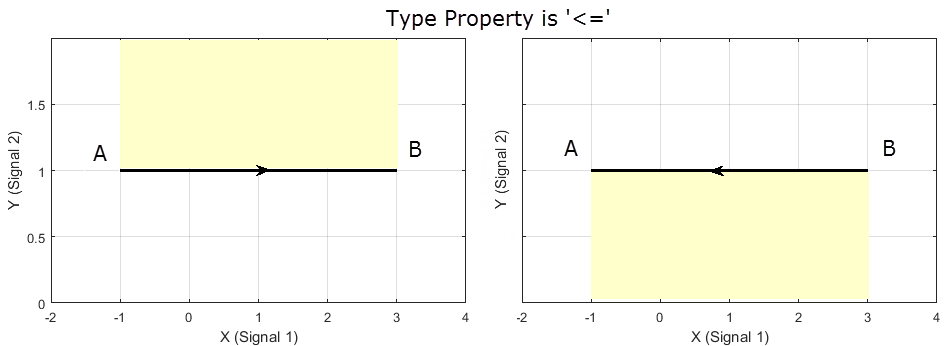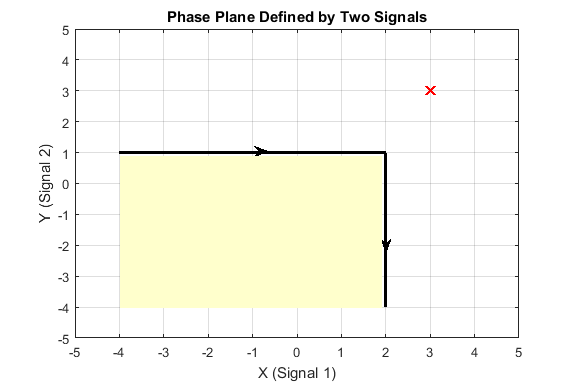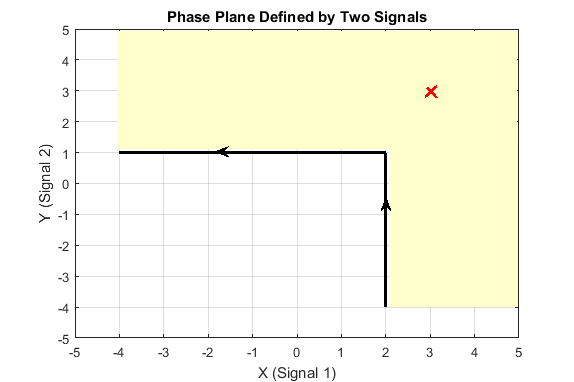Documentation

sdo.requirements.PhasePlaneRegion class

Package: sdo.requirements
Superclasses:

Impose region bound on phase plane trajectory of two signals

Description

Use the sdo.requirements.PhasePlaneRegion object to impose a region bound on the phase plane trajectory of two signals in a Simulink® model. The phase plane trajectory is a plot of the two signals against each other. In the object, you can specify the bounded region as a single edge, or multiple piecewise-linear edges. You specify the starting and ending x and y coordinates of the bound edges, where the X-Y plane is the phase plane defined by the two signals. You also specify whether you require the trajectory of the two signals to lie inside or outside the bounded region specified by the edges.

You can use the object as an input to your cost function, and use the evalRequirement command in the cost function to evaluate whether your test signals satisfy the specified requirement. You can then use the cost function and sdo.optimize to perform parameter estimation or response optimization, subject to the satisfaction of the specified requirement. If you are performing sensitivity analysis, after you generate parameter samples, you can use the cost function and sdo.evaluate to evaluate the requirement for each generated sample.

Construction

requirement = sdo.requirements.PhasePlaneRegion creates an sdo.requirements.PhasePlaneRegion requirement object and assigns default values to its properties. Use dot notation to customize the properties of the object, except bound edges. To specify the bound edges simultaneously, use the set command. Use the evalRequirement command to evaluate whether test signals satisfy the specified requirement.

requirement = sdo.requirements.PhasePlaneRegion(Name,Value) creates the requirement object with additional options specified by one or more Name,Value pair arguments. Name is a property name and Value is the corresponding value. Name must appear inside single quotes (''). You can specify several name-value pair arguments in any order as Name1,Value1,...,NameN,ValueN.

Input Arguments

Name-Value Pair Arguments

Specify optional comma-separated pairs of Name,Value arguments. Name is the argument name and Value is the corresponding value. Name must appear inside quotes. You can specify several name and value pair arguments in any order as Name1,Value1,...,NameN,ValueN.

Use Name,Value arguments to specify properties of the requirement object during object creation. For example, requirement = sdo.requirements.PhasePlaneRegion('OpenEnd',[1 1]) creates an sdo.requirements.PhasePlaneRegion object and specifies that the first and last edge of the bound extend to infinity.

Properties

expand all

X-coordinates of edges that define the bounded region, specified as an n-by-2 array with finite values, where n is the number of edges in the bound. Each row of BoundX specifies the starting and ending x-coordinate values of an edge. The number of rows must match the number of rows in the BoundY property, which specifies the y-coordinates of the edges.

You must specify the BoundX and BoundY properties simultaneously, either using Name,Value arguments during object construction, or using the set command after object construction.

Data Types: double

Y-coordinates of edges that define the bounded region, specified as an n-by-2 array with finite values, where n is the number of edges in the bound. Each row of BoundY specifies the starting and ending y-coordinate values of an edge. The number of rows must match the number of rows in the BoundX property, which specifies the x-coordinates of the edges.

You must specify the BoundX and BoundY properties simultaneously, either using Name,Value arguments during object construction, or using the set command after object construction.

Data Types: double

Requirement description, specified as a character vector.

Example: 'Requirement 1 for myModel.'

Data Types: char

Extension of first and last bound edges to infinity, specified as a 1-by-2 logical array.

If the first element of OpenEnd is true, the beginning of the first edge in the piecewise-linear bound extends to infinity. If the second element of OpenEnd is true, the end of the last edge in the piecewise-linear bound extends to infinity.

For an example, see Create a Phase Plane Region Bound With Edge Extending to Infinity.

Data Types: logical

Name of requirement, specified as a character vector.

Example: 'Requirement1'

Data Types: char

Type of bound, specified as one of the following:

• '<=' — The out-of-bound region is always to the left of each edge, where the forward direction is the direction of creation of the edge.

For example, consider a single-edge bound between the point A located at (-1,1) and point B at (3,1). Suppose you specify the coordinates of point A before you specify point B. That is, you specify BoundX as [-1 3] and BoundY as [1 1]. The black arrow in the left plot shows the direction of creation of the edge, and the yellow region is the out-of-bound region when Type is '<='. Now suppose you instead specify point B before point A. That is, you specify BoundX is [3 -1] and BoundY is [1 1]. The right plot shows that the out-of-bound region is now below the edge because the direction of creation of the edge has reversed.• '>=' — The out-of-bound region is always to the right of each edge.

For the single-edge example, suppose that you specify the coordinates of point A before you specify point B. In the left plot you can see that the yellow out-of-bound region is below the edge when Type is '>='. This is because the yellow region is to the right of the edge, in the direction of creation of the edge. If you instead specify point B before point A, the right plot shows that the out-of-bound region is now above the edge.For an example with multiple bound edges, see Specify Bounding Region With Multiple Edges and Evaluate the Requirement.

Data Types: char

Methods

 evalRequirement Evaluate satisfaction of piecewise-linear bound on phase plane trajectory of two signals

Copy Semantics

Handle. To learn how handle classes affect copy operations, see Copying Objects (MATLAB).

Examples

collapse all

Create a phase plane region requirement object with default properties. The object specifies a piecewise-linear bound on the phase plane trajectory of two signals. The phase plane is the X-Y plane defined by the two signals.

Requirement = sdo.requirements.PhasePlaneRegion;

Specify a piecewise-linear bound with two edges. The (x,y) coordinates for the beginning and end of the first edge are (1,1) and (2,1). The second edge extends from (2,1) to (2,0). You must specify the BoundX and BoundY properties simultaneously.

set(Requirement,'BoundX',[1 2; 2 2],'BoundY',[1 1; 1 0])

Specify that the beginning of the first edge extends to infinity.

Requirement.OpenEnd = [1 0];

The first edge now extends from (-Inf,1) to (2,1).

You can now use the evalRequirement command to evaluate whether test data from two signals satisfy the requirement.

Create a requirement object to specify a piecewise-linear bound on the phase plane trajectory of two signals. The bound has two edges. The first edge extends from (-4,1) to (2,1). The second edge extends from (2,1) to (2,-4).

Requirement = sdo.requirements.PhasePlaneRegion('BoundX',[-4 2; 2 2],...
'BoundY',[1 1; 1 -4]);

Specify the bound type as '>='.

Requirement.Type = '>=';

The plot below shows the bounding edges in black. The arrows indicate the direction in which the edges were specified. When you specify the Type property as '>=', the out-of-bound area is always to the right of each edge, where the forward direction is the direction of creation of the edge. As a result, the out-of bound region is the yellow shaded area, and a trajectory point located at (3,3) is in the bounded region.Evaluate the requirement for the phase plane trajectory point located at (3,3).

Evaluation = evalRequirement(Requirement,[3 3])
Evaluation = -0.6389

evalRequirement returns a negative number, indicating the requirement is satisfied.

Now create the requirement by changing the order of specification of the edges.

set(Requirement,'BoundX',[2 2; 2 -4],'BoundY',[-4 1;1 1]);The plot shows that the edges were created in the opposite order. So, even though the requirement type is still '>=', the out-of-bound region, which is always to the right of the edges, is now flipped.

Evaluate the requirement.

Evaluation = evalRequirement(Requirement,[3 3])
Evaluation = 0.1087

A positive Evaluation value indicates the requirement has been violated. Thus, for the same requirement type, the trajectory point at (3,3) is out of bounds when the edges are defined in the reverse order.